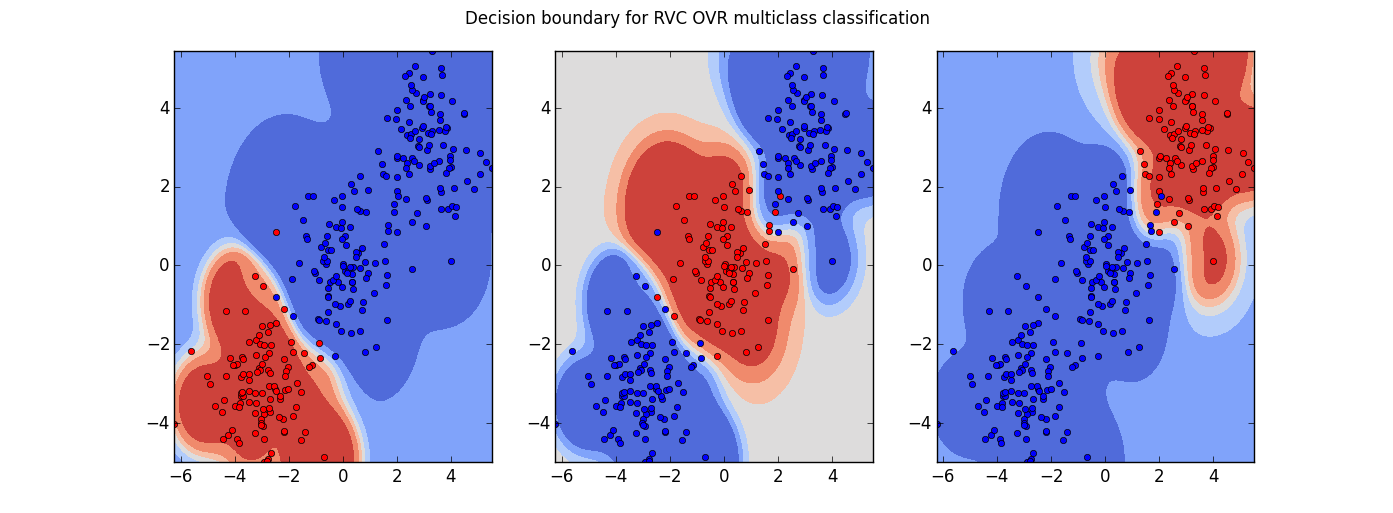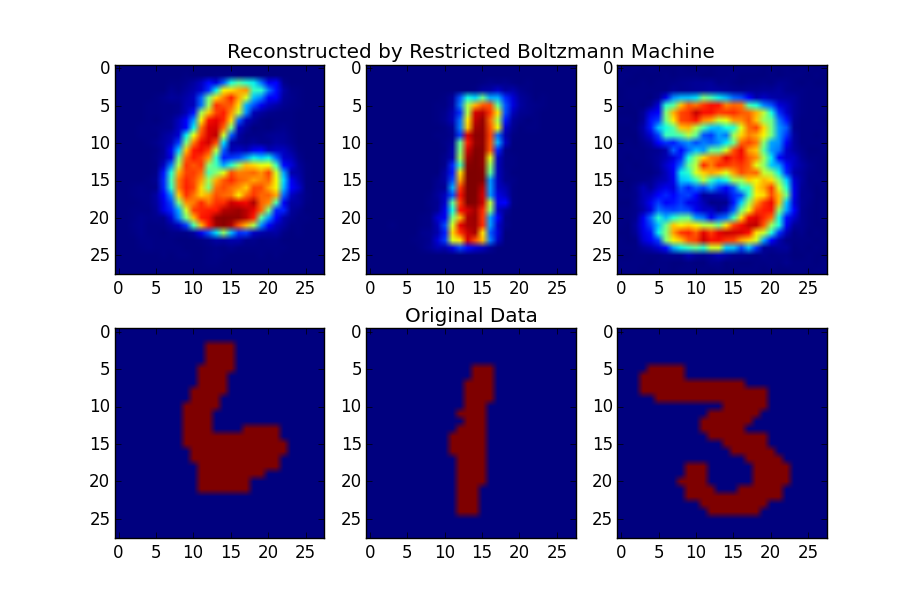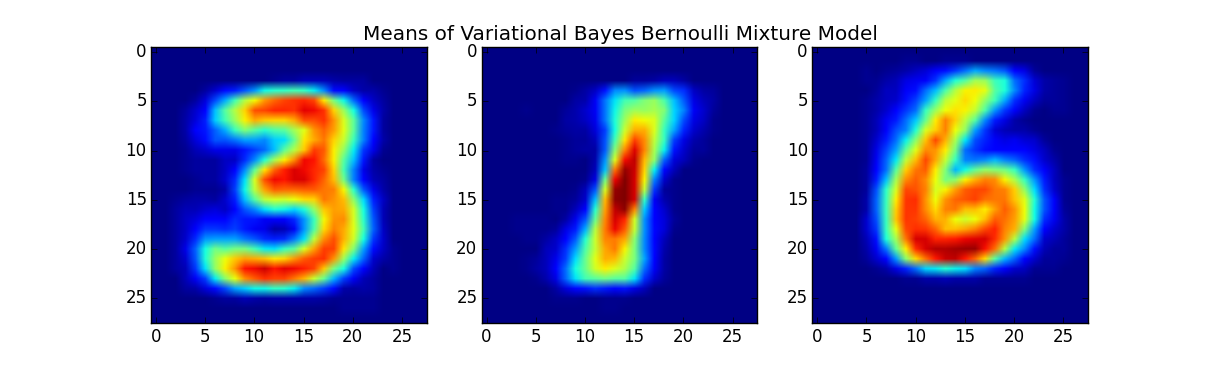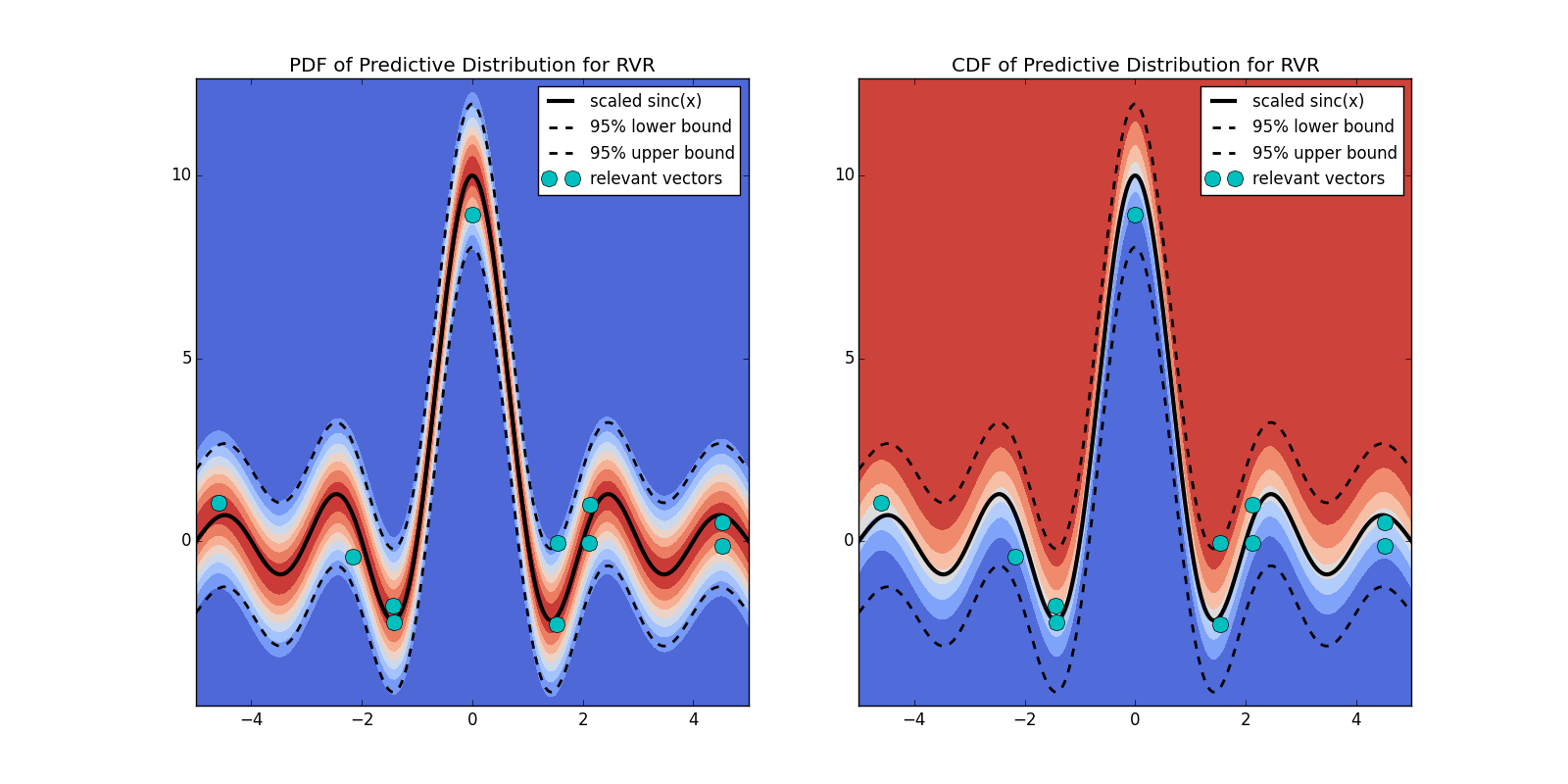# Sklearn Bayes

Python package for Bayesian Machine Learning with scikit-learn API
Alternatives To Sklearn Bayes
Pyro8,131187412 days ago34July 29, 2023242apache-2.0Python
Deep universal probabilistic programming with Python and PyTorch
Deeplearning2,347
3 years ago3mitPython
Python for《Deep Learning》，该书为《深度学习》(花书) 数学推导、原理剖析与源码级别代码实现
Gen.jl1,745
12 days ago155apache-2.0Julia
A general-purpose probabilistic programming system with programmable inference
Orbit1,72816 days ago21January 29, 202365otherPython
A Python package for Bayesian forecasting with object-oriented design and probabilistic models under the hood.
Bayesian Machine Learning1,587
8 months ago4apache-2.0Jupyter Notebook
Notebooks about Bayesian methods for machine learning
Imodels1,17548 days ago43August 22, 202326mitJupyter Notebook
Interpretable ML package 🔍 for concise, transparent, and accurate predictive modeling (sklearn-compatible).
Nips2017913
6 years ago
A list of resources for all invited talks, tutorials, workshops and presentations at NIPS 2017
Bayeslite828
3 years ago193apache-2.0Python
BayesDB on SQLite. A Bayesian database table for querying the probable implications of data as easily as SQL databases query the data itself.
Emukit533221 days ago7September 22, 202141apache-2.0Python
A Python-based toolbox of various methods in decision making, uncertainty quantification and statistical emulation: multi-fidelity, experimental design, Bayesian optimisation, Bayesian quadrature, etc.
Bayesiandeeplearning Survey449
2 months ago
Bayesian Deep Learning: A Survey
Alternatives To Sklearn Bayes
Select To Compare

Alternative Project Comparisons

## Python package for Bayesian Machine Learning with scikit-learn API``````pip install https://github.com/AmazaspShumik/sklearn_bayes/archive/master.zip
``````

### Algorithms

• ARD Models
• Relevance Vector Regression (version 2.0) code, tutorial
• Relevance Vector Classifier (version 2.0) code, tutorial
• Type II Maximum Likelihood ARD Linear Regression code
• Type II Maximum Likelihood ARD Logistic Regression code, tutorial
• Variational Relevance Vector Regression code
• Variational Relevance Vector Classification code, tutorial
• Decomposition Models
• Restricted Boltzmann Machines (PCD-k / CD-k, weight decay, adaptive learning rate) code, tutorial
• Latent Dirichlet Allocation (collapsed Gibbs Sampler) code, tutorial
• Linear Models
• Empirical Bayes Linear Regression code, tutorial
• Empirical Bayes Logistic Regression (uses Laplace Approximation) code, tutorial
• Variational Bayes Linear Regression code, tutorial
• Variational Bayes Logististic Regression (uses Jordan local variational bound) code, tutorial
• Mixture Models
• Variational Bayes Gaussian Mixture Model with Automatic Model Selection code, tutorial
• Variational Bayes Bernoulli Mixture Model code, tutorial
• Dirichlet Process Bernoulli Mixture Model code
• Dirichlet Process Poisson Mixture Model code
• Variational Multinoulli Mixture Model code
• Hidden Markov Models
• Variational Bayes Poisson Hidden Markov Model code, demo
• Variational Bayes Bernoulli Hidden Markov Model code
• Variational Bayes Gaussian Hidden Markov Model code, demo

### Contributions:

There are several ways to contribute (and all are welcomed)

`````` * improve quality of existing code (find bugs, suggest optimization, etc.)
* implement machine learning algorithm (it should be bayesian; you should also provide examples & notebooks)
* implement new ipython notebooks with examples
``````Popular Bayesian Projects
Popular Machine Learning Projects
Popular Machine Learning Categories
Related Searches

Get A Weekly Email With Trending Projects For These Categories
No Spam. Unsubscribe easily at any time.
Python
Jupyter Notebook
Machine Learning
Tutorial
Bayesian
Scikit Learn